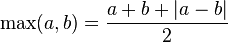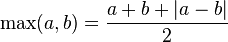# Definition of max and min

I found in the wiki a definition for the max of 2 numbers:https://en.wikipedia.org/wiki/Ramp_function

But is definition is only for 2 numbers, how would be the definition for 3 numbers?

Also, which is the definition of minimum function?

mathman
min(a,b) = (a+b-|a-b|)/2.

I can't see anything analogous for 3 numbers.

Mark44
Mentor
I found in the wiki a definition for the max of 2 numbers:https://en.wikipedia.org/wiki/Ramp_function

But is definition is only for 2 numbers, how would be the definition for 3 numbers?
Maybe you could figure one out.

In any case, being able to calculate the maximum of two numbers is much more important than being able to calculate the maximum of three numbers. You can do this by picking any two of them, and calculating which of the two is larger. Then compare that number with the third number. Whichever number is larger is the maximum of the three numbers. You can extend this idea to four, five, ..., n numbers.
Also, which is the definition of minimum function?
$$Min(a, b) = \frac{a + b - |a - b|}{2}$$

The formula for the maximum of two numbers is straightforward if you look at the geometry.
$$Max(a, b) = \frac{a + b + |a - b|}{2} = \frac{a + b}{2} + \frac{|a - b|}{2}$$
The first fraction on the right is the number that is midway between the points a and b. The second fraction is half the distance between the two points. From the midway point, if you add half the distance between the two points, you end up at whichever point is on the right; i.e., the larger number.

In the formula I wrote for the minimum, the only difference is that we are subtracting half the distance between the two points, which gets us to the point on the left - the smaller number.

•1 person
Curious3141
Homework Helper
I found in the wiki a definition for the max of 2 numbers:https://en.wikipedia.org/wiki/Ramp_function

But is definition is only for 2 numbers, how would be the definition for 3 numbers?

Can't you recurse? max(a,b,c) = max(max(a,b),c).

Those formulas not says too when a complex number is greater another?

Curious3141
Homework Helper
Those formulas not says too when a complex number is greater another?

Complex numbers are not orderable. It is meaningless to speak of one complex number being greater than or less than another.

You can only say that about their magnitudes or arguments (within a defined range of angle measure).

jbriggs444
Homework Helper
Complex numbers are not orderable. It is meaningless to speak of one complex number being greater than or less than another.

Note that when we say that the complex numbers are not orderable, it is not that one cannot come up with a sorting order for them. It is that one cannot come up with a sorting order that is consistent with the way the operations of addition and multiplication are expected to work with an order.

In particular, you want to be able to say that if a > 0 and b > 0 then ab > 0. But if you take a=b=i then ab = -1. That then requires that -1 > 0.

In other words, the complex numbers do not form an "ordered field". http://en.wikipedia.org/wiki/Ordered_field

Last edited:
Curious3141
Homework Helper
Note that when we say that the complex numbers are not orderable, it is not that one cannot come up with a sorting order for them. It is that one cannot come up with a sorting order that is consistent with the way the operations of addition and multiplication are expected to work with an order.

In particular, you want to be able to say that if a > 0 and b > 0 then ab > 0. But if you take a=b=i then ab = -1. That then requires that -1 > 0.

In other words, the complex numbers do not form an "ordered field". http://en.wikipedia.org/wiki/Ordered_field

I just saw this. I'm aware of the concept of an ordered field, I just didn't want to overcomplicate things when it's not really relevant here.